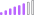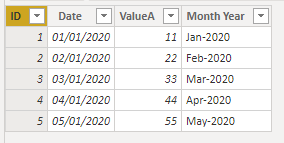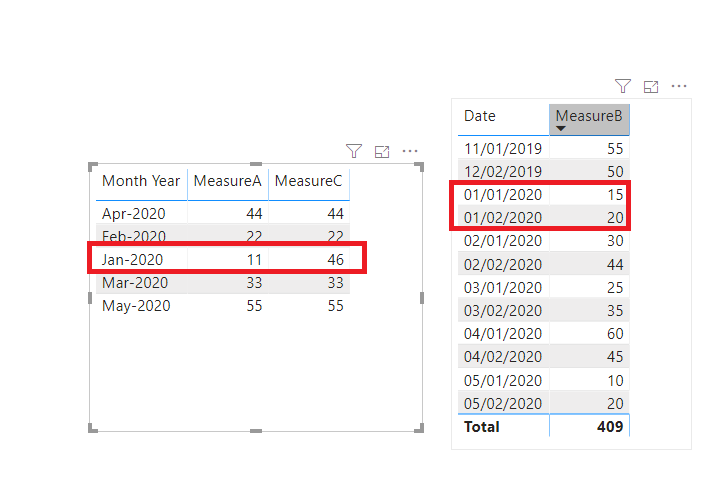cancel
Showing results for
Did you mean:Post Patron

## Condition a measure by what is in scope in the graph

I've got an histogram with MeasureA=SUM([ValueA]) by Year and Month based on fields on TableA.

I've got TableB with field [ValueB] and MeasureB=SUM([ValueB]).

I would like to create a MeasureC=MeasureA+MeasureB where MeasureB adds its value on the histogram only on current year bar or current year and current month.

Is it possible?

1 ACCEPTED SOLUTIONCommunity Support

Hi, @AGo

Table A:Table B:You may create measures as follows.

``````MeasureA = SUM('Table A'[ValueA])

MeasureB = SUM('Table B'[ValueB])

MeasureC =
var _currentmonth = MONTH(TODAY())
var _currentyear = YEAR(TODAY())
var _date = MAX('Table A'[Date])

return
IF(
MONTH(_date) = _currentmonth&&YEAR(_date)=_currentyear,
CALCULATE(
[MeasureA],
FILTER(
ALL('Table A'),
'Table A'[Date].[MonthNo] = _currentmonth&&'Table A'[Date].[Year]=_currentyear
)
)+
CALCULATE(
[MeasureB],
FILTER(
ALL('Table B'),
'Table B'[Date].[MonthNo] = _currentmonth&&'Table B'[Date].[Year]=_currentyear
)
),
[MeasureA]
) ``````

Result:Best Regards

Allan

If this post helps, then please consider Accept it as the solution to help the other members find it more quickly.

2 REPLIES 2Community Support

Hi, @AGo

Table A:Table B:You may create measures as follows.

``````MeasureA = SUM('Table A'[ValueA])

MeasureB = SUM('Table B'[ValueB])

MeasureC =
var _currentmonth = MONTH(TODAY())
var _currentyear = YEAR(TODAY())
var _date = MAX('Table A'[Date])

return
IF(
MONTH(_date) = _currentmonth&&YEAR(_date)=_currentyear,
CALCULATE(
[MeasureA],
FILTER(
ALL('Table A'),
'Table A'[Date].[MonthNo] = _currentmonth&&'Table A'[Date].[Year]=_currentyear
)
)+
CALCULATE(
[MeasureB],
FILTER(
ALL('Table B'),
'Table B'[Date].[MonthNo] = _currentmonth&&'Table B'[Date].[Year]=_currentyear
)
),
[MeasureA]
) ``````

Result:Best Regards

Allan

If this post helps, then please consider Accept it as the solution to help the other members find it more quickly.Resident Rockstar

Well yes but that really depends on your datastructure to have an exact answer. It will be somewhere along the lines of this:

``````MeasureC =
VAR _curSelectedYear = YEAR(MAX(Table[Date]))
VAR _curSelectedMonth = MONTH(MAX(Table[Date]))
VAR _currentYear = YEAR(TODAY())
VAR _currentMonth = MONTH(TODAY())
VAR _toAdd = IF(_curSelectedYear = _currentYear && _curSelectedMonth = _currentMonth, [MeasureB], 0)
RETURN

SOmething like this?

Kind regards

Djerro123

-------------------------------

If this answered your question, please mark it as the Solution. This also helps others to find what they are looking for.

Keep those thumbs up coming! 🙂

Proud to be a Super User!Announcements#### Exclusive opportunity for Women!

Join us for a free, hands-on Microsoft workshop led by women trainers for women where you will learn how to build a Dashboard in a Day!#### Power Platform Conference-Power BI and Fabric Sessions

Join us Oct 1 - 6 in Las Vegas for the Microsoft Power Platform Conference.Top Solution Authors
Top Kudoed Authors
Users online (2,902)